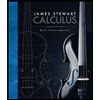# Verify that the Divergence Theorem is true for the vector field F on the region E. F(x, y, z) =3x i + xy j + 2xz k, E is the cube bounded by the planes x=0, x = 1, y=0, y= 1, z=0, and z=1

Question

Verify that the Divergence Theorem is true for the vector field F on the region E.

F(x, y, z) =3x i + xy j + 2xz k, E is the cube bounded by the planes x=0, x = 1, y=0, y= 1, z=0, and z=1

Expert Solution

### Want to see the full answer?

Check out a sample Q&A hereStudents who’ve seen this question also like:Calculus: Early Transcendentals
8th Edition
ISBN: 9781285741550
Author: James Stewart
Publisher: Cengage Learning